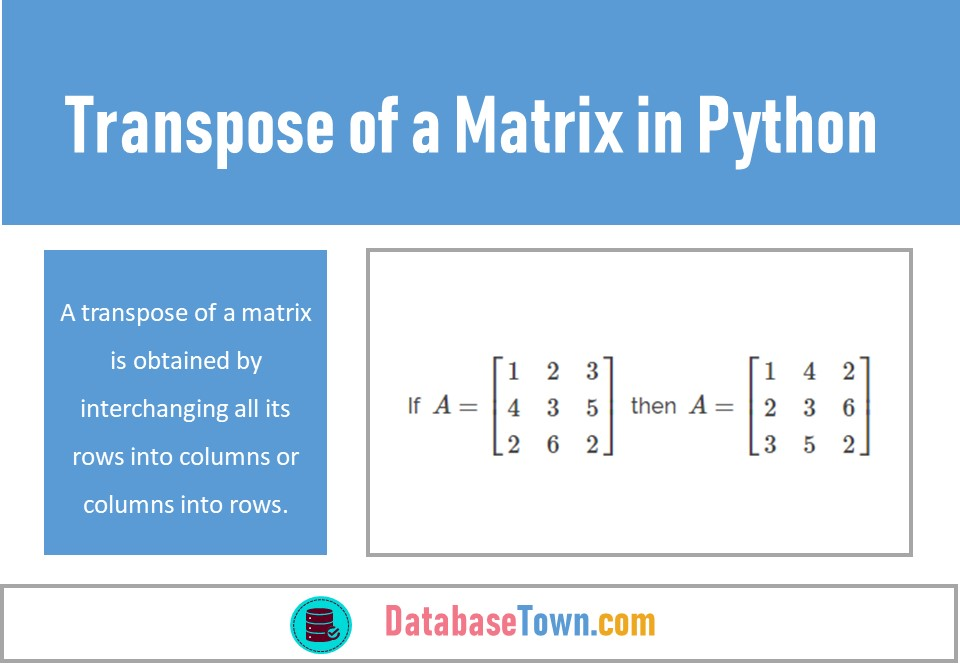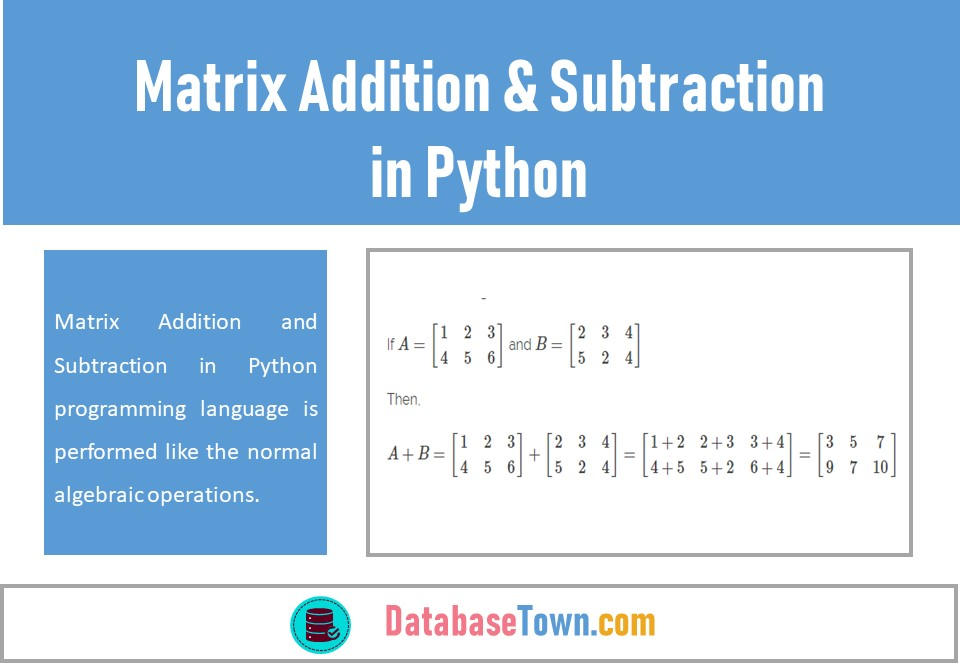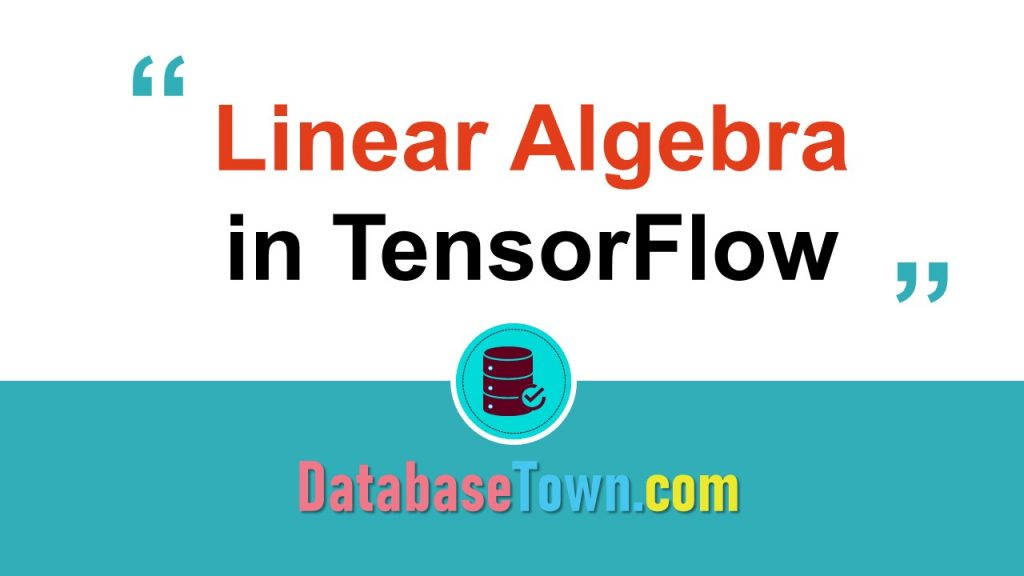# Mathematics for Data Science## Transpose of a Matrix in Python

A transpose of a matrix is obtained by interchanging all its rows into columns or columns into rows. It is denoted by $$\displaystyle {{A}^{t}}$$ or $$\displaystyle {{A}^{‘}}$$. For example, If  $$\displaystyle A=\left[ {\begin{array}{*{20}{c}} 1 & 2 & 3 \\ 4 & 3 & 5 \\ 2 & 6 & 2 \end{array}} \right]\,$$ then  \(\displaystyle A=\left[ …## Matrix Addition and Subtraction in Python

Matrix Addition and Subtraction in Python programming language is performed like the normal algebraic operations. Before discussing these operations, it is necessary to introduce a bit about Algebra which has been taken from the Arabic word Al-Jabar, afterward, this word turned into Algebra. Algebra is a branch of Mathematics that provides an easy solution to …## Linear Algebra in TensorFlow (Scalars, Vectors & Matrices)

Linear Algebra in TensorFlow: TensorFlow is open source software under Apache Open Source license for dataflow which is frequently being used for machine learning applications like deep-neural-network to improve the performance of search engines, such as, Google, image captioning, recommendation and translation. For example, when a user types a keyword in Google’s search bar, it …## Scalars, Vector and Matrices in Python (Using Arrays)

Arrays in python, are frequently used to work with scalars, vectors and matrices, a topic of today’s post. This post is continuation of linear algebra for data science. We use NumPy, a library for the python programming which allows us to work with multidimensional arrays and matrices along with a large collection of high-level mathematical …## Linear Algebra for Data Science

Linear Algebra for Data Science and machine learning is very essential as the concepts of linear algebra are used to understand the working of algorithms. In this post, we are going to discuss the basic concepts of linear algebra. Why Linear Algebra? Enormous datasets mostly contain hundreds to a large number of individual data objects. …